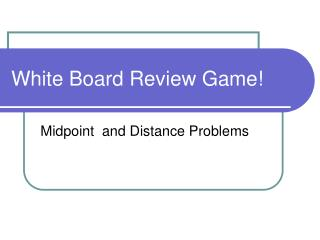DownloadDownload PresentationWhite Board Review Game!

# White Board Review Game!

Download Presentation## White Board Review Game!

- - - - - - - - - - - - - - - - - - - - - - - - - - - E N D - - - - - - - - - - - - - - - - - - - - - - - - - - -
##### Presentation Transcript

1. White Board Review Game! Midpoint and Distance Problems

2. Midpoint Formula

3. 1) (1, 2) (5, 4) 2) (-1, 2) (7, 4) 3) (-3, 3) (2, -2) (3, 3) (3, 3) ( -½, ½) Find the midpt between the points

4. Find the midpt between the points • 4) (-1, 1) (-4, -4) • 5) (-4, 0) (-1, -5) • 6) (-4, -3) (-1, -5)

5. Distance Formula Remember: First subtract inside ( ) Then square each ‘piece’ Next add the ‘pieces’ Finally, take the square root

6. Find the Distance Specified • 7) A (3, 0) B (-1, 2) • 8) C (-5, 4) D (-2, 3)

7. Find the Distance Specified • 9) A (5, -2) B (1, 7) • 10) C (-3, 6) D (1, 11)

8. Solve the equation for y • 11) 5x + y = 10 • 12) 6x + 3y = 12 • Bonus: 4x – 8y = 24

9. Quiz Tomorrow! • Topics • Plotting points • Graphing from a table • Solving an equation for y • Graphing Horizontal and Vertical Lines • Distance • Midpoint